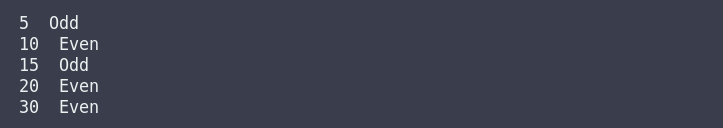# cpp programming exercise with solution

Write a c++ program in which you have to initialize an unsorted array of five integer numbers then sort that array and also print whether the number is even or odd.cpp programming exercise with solution

Note below program logic is just for sorting five elements array

#include <iostream>
using namespace std;

int main()
{
int arr = {30, 5, 10, 15, 20};
// loop for print lowest elements first
for (int i = 0; i < 5; i++)
{
if (arr[i] < arr[i+1])
{
cout<<arr[i]<<" ";
if (arr[i]%2 == 0)
{
cout<<" Even "<<endl;
}

else
{
cout<<" Odd "<<endl;
}
}
}
// loop for print highest elements at last
for (int i = 0; i < 5; i++)
{
if (arr[i] > arr[i+1])
{
cout<<arr[i]<<" ";
if (arr[i]%2 == 0)
{
cout<<" Even "<<endl;
}

else
{
cout<<" Odd "<<endl;
}
}

}

return 0;
}